|

# 北欧木居开幕——北京老工业区飞来瑞典小木屋

9月28日，受北京国际设计周751国际设计节邀请，由北欧可持续设计协会（SSDA）策划、北京水木一方规划设计有限公司承办的北欧木居（Nordic Space）于751D·PARK北京时尚设计广场炉区北广场隆重开幕，展览以“手工与可持续设计”为主题，汇集近20个来自瑞典和北欧的本土品牌和机构参与。瑞典驻华大使馆新闻参赞Andre Mkandawire，北欧木居活动策展人、SSDA执行长Soifa He，瑞典著名产品设计和室内建筑师Jon Eliason，北京迪百可文化发展有限责任公司总经理张军元，苏州昆仑绿建总经理助理张琴等人出席了开幕式。北欧木居（Nordic Space）打造由6个形态各异的木房组成的原生态北欧生活体验馆，以木为主要元素，结合建筑与家居，传统与现代，试图还原“林子里的北欧人”的真实生活。参加本次展览的品牌和机构包括多个瑞典皇家御用品牌如达拉木马、天堂陶瓷，海丝腾顶级床具等，同时有著名艺术家和设计师Inger Åberg艺术手工壁毯、Jon Eliason的玻璃作品等。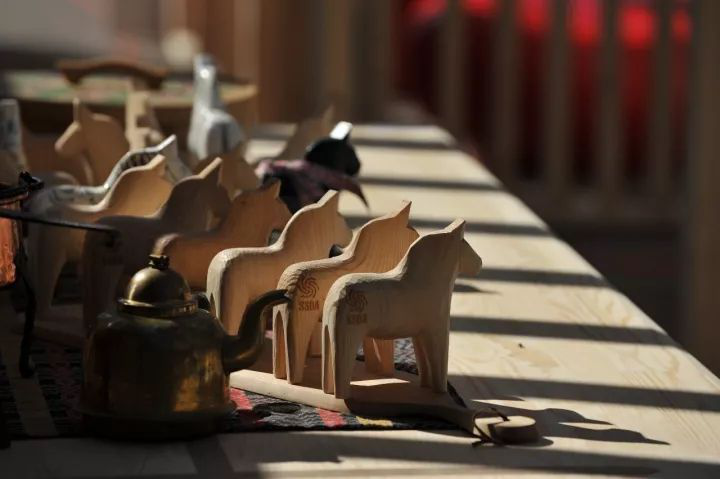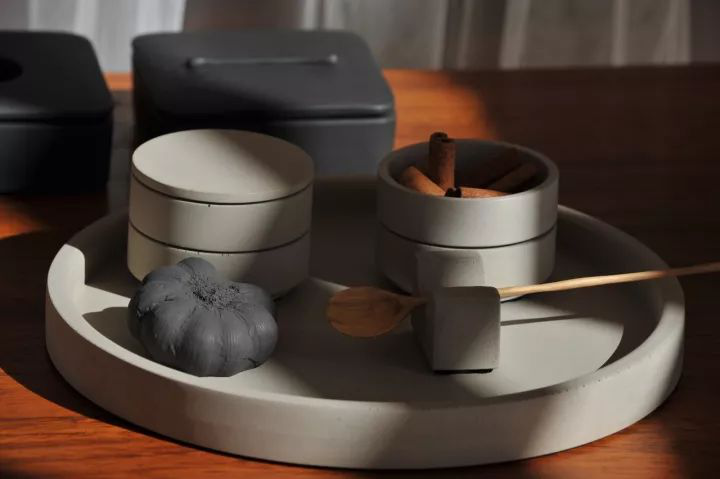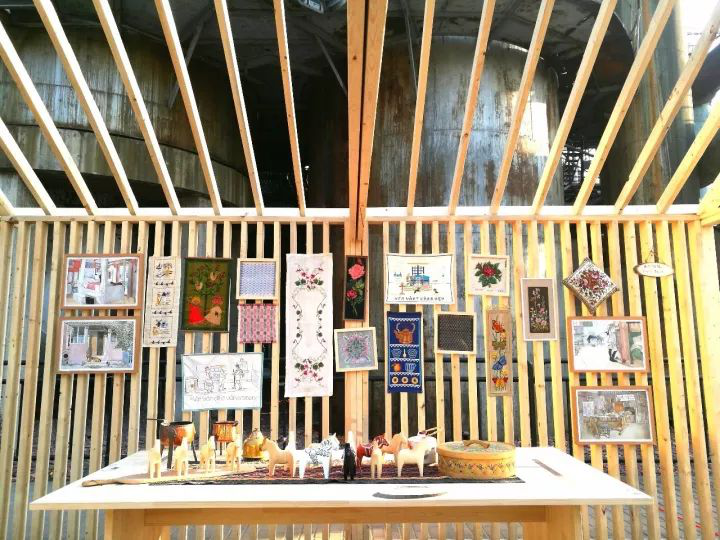Sofia表示，本次展出为大家展现了瑞典本土的居家环境、全面可持续设计理念和健康生活方式。展览主题是木屋和手工。木，是与人类最为接近的材料，瑞典70%的土地由森林覆盖，瑞典现在建筑工艺能够建造八层全木楼房。而在一千多年前的中国，木结构建筑工艺在世界上都处于领先地位。

木料作为唯一可再生建筑材料，充分代表着绿色环保的理念。木料也易于设计师灵活表达个性设计和体现人性化服务。现在国际上追求返璞归真，木居让人联想到宁静的田园生活，即使在繁华都市也能感受到土地的亲切。作为海外华人，经过多年思考和探索，Sofia在2014年成立了北欧可持续设计协会，旨在将北欧优秀设计理念、价值观念和理论学说传入中国，让国人足不出户就能亲身体验。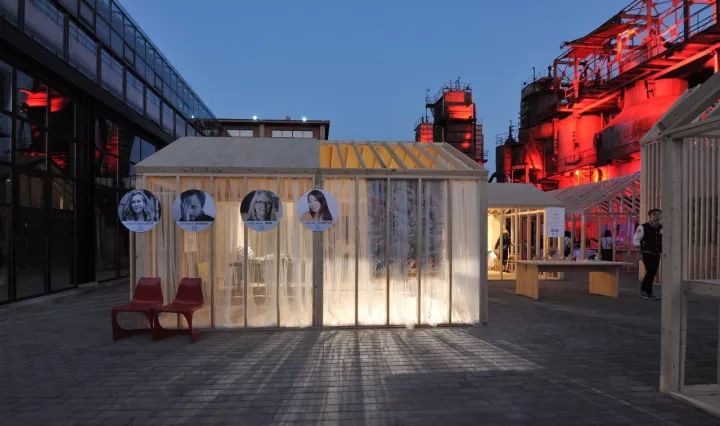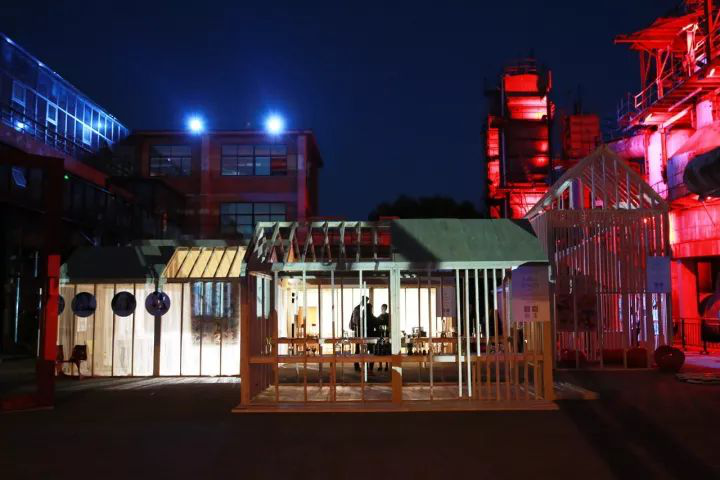瑞典驻华大使馆新闻参赞Andre Mkandawire认为北欧木居（Nordic Space）是对瑞典文化传达的启动。他认为Sofia作为个体，所做的一切都是令人惊叹的。展览像一个橱窗展现了瑞典的生活美学。他很开心能够作为瑞典驻华大使馆代表见证这里点点滴滴的变化。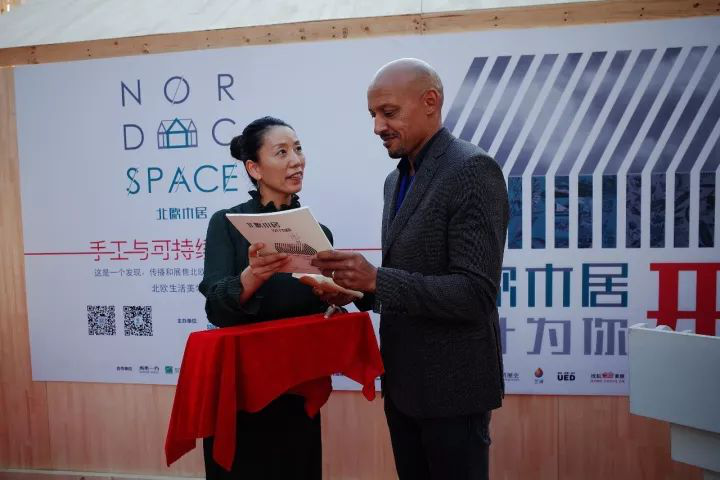（左：Sofia 右：Andre）

Jon Eliason表示打造北欧木居的灵感来自瑞典本土文化元素，瑞典传统乡村居民会世代住在木屋里，朝夕相处，亲密无间，这正是瑞典能够走到今天的基础。对于瑞典人来说，手工和木工都是极为重要的。他想把瑞典传统小村庄带入北京，让木材与钢筋水泥相融对比产生奇妙视觉效果。从内到外，通透结合。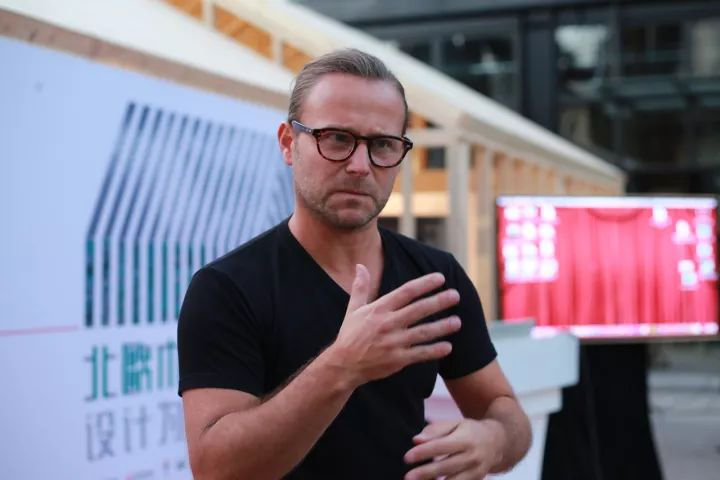（Jon Eliason）

SSDA将瑞典皇储大婚时打造的限量达拉木马拍卖赠予天下溪乡土教材项目，负责人王小平老师希望孩子们通过乡土教材继承文化艺术，也希望中国会有越来越多的“达拉木马之乡”。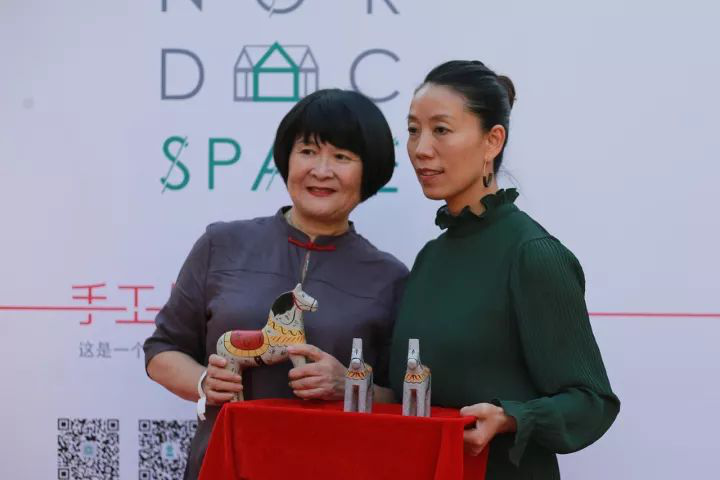(左：王小平老师 右：Sofia)

开幕式上，Sofia宣布了首批北欧可持续设计协会公益大使名单，包括上海微建（Vjian）建筑空间设计有限公司董事长、首席设计师宋微建，场域建筑（北京）工作室主持建筑师、城市研究者梁井宇，智立方集团董事长兼CEO杨石头，北京建筑大学大师工作室之王刚工作室主持建筑师王刚，四面田工作室创始合伙人王蔚，设计腕儿（Designwire）创始人兼主编马海金，《VISION青年视觉》设计总监孙初和影像艺术家杨国伟。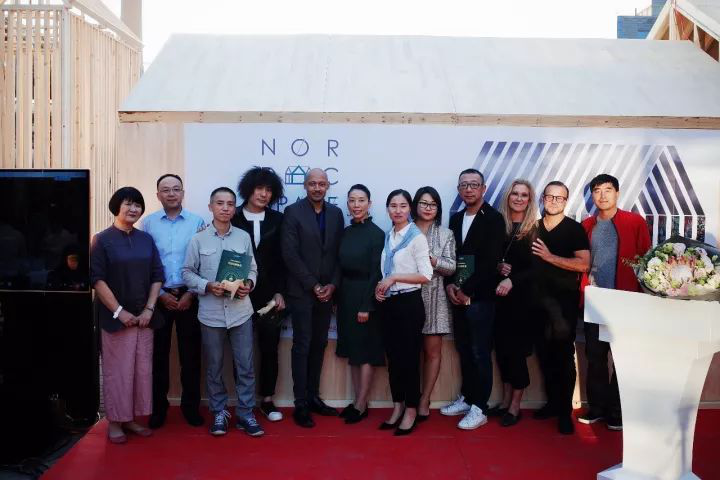（公益大使合影）

9月30日，瑞典驻华大使Anna Lindstedt和瑞典驻华大使馆文化参赞Mathias Lafolie一行来访北欧木居（Nordic Space）展。

Sofia带领大使一行人参观展区，讲述了布展经过和理念，瑞典设计师Jon Eliason和Anna Christians也分别详细介绍了自己的产品。随后，Sofia邀请嘉宾在主展厅就坐，观看了北欧木居(Nordic Space)搭建施工布展的整个流程视频。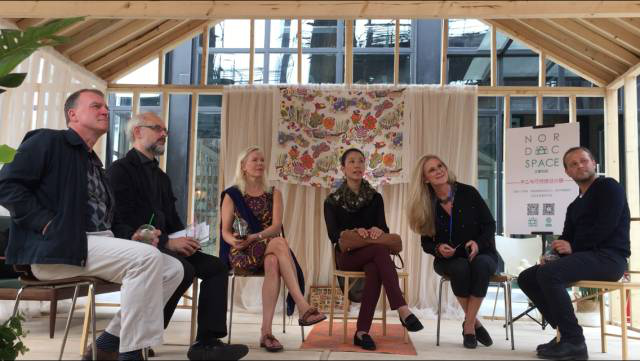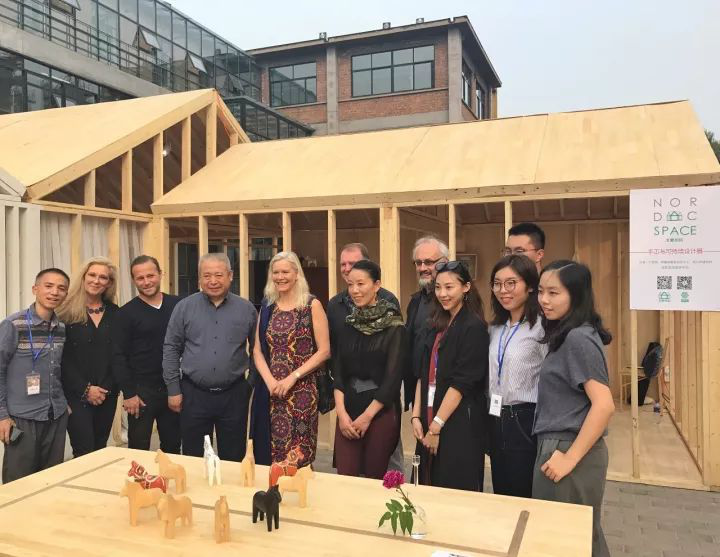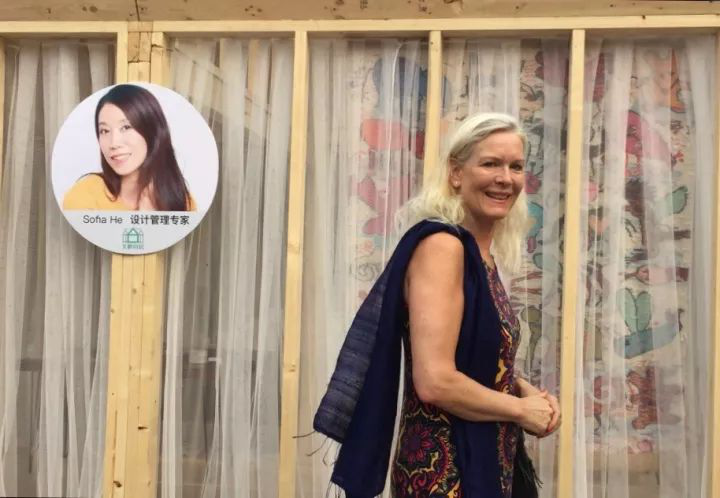（瑞典驻华大使Anna Lindstedt）

10月2日，北京设计周组委会副主任、策划总监曾辉参观北欧木居（Nordic Space）展，充分肯定了这次展览在中国和北欧之间手工设计文化交流的重要意义，并邀请SSDA继续参与明年的国际设计周，把中国传统手工艺与北欧设计相结合，推广手工和可持续设计理念。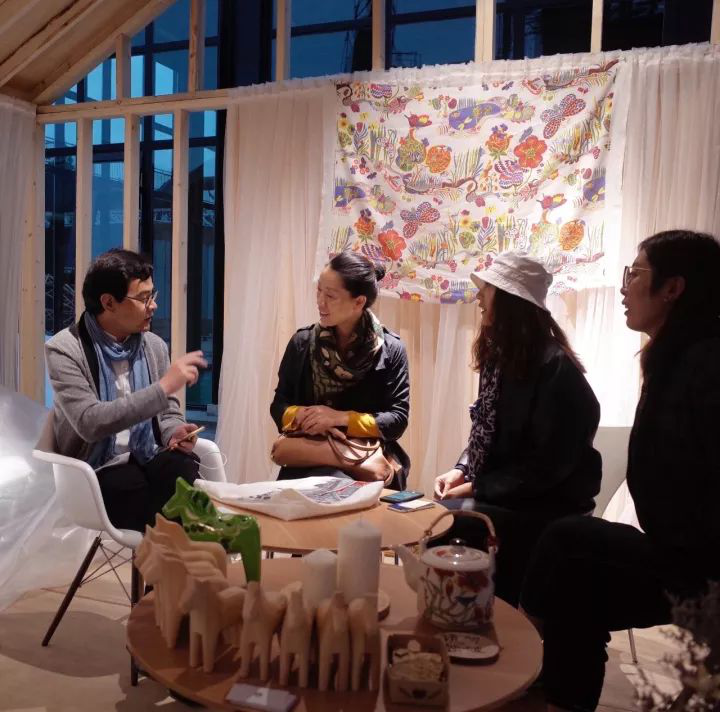（左一：曾辉左二：Sofia）

本次展览从9月27日-10月6日为期10天，活动期间有北欧设计课堂，框住北欧，Sofia带你游北欧，北欧FIAK美食体验，以及留住时光北欧旧事集等精彩纷呈的活动。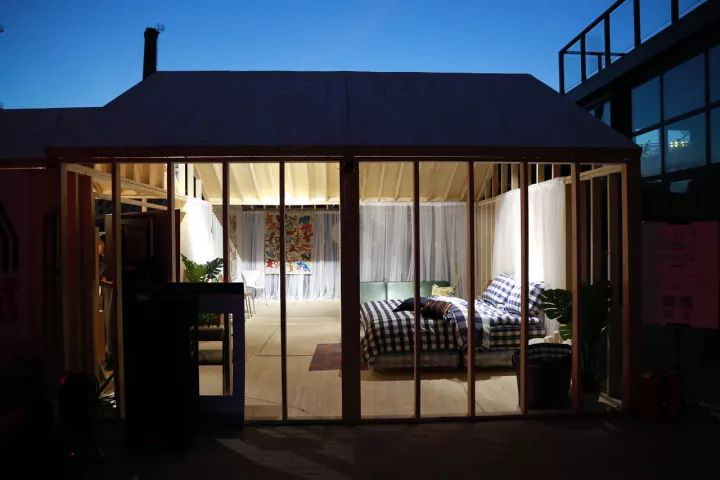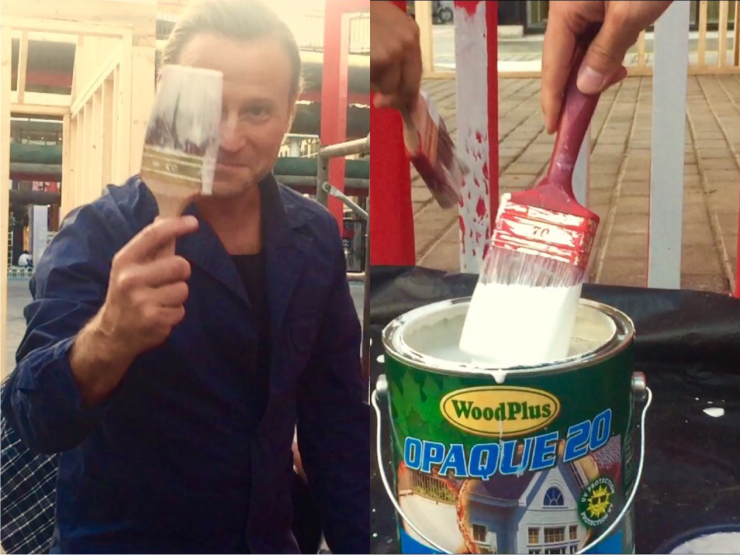（北欧木居涂料支持：Woodplus）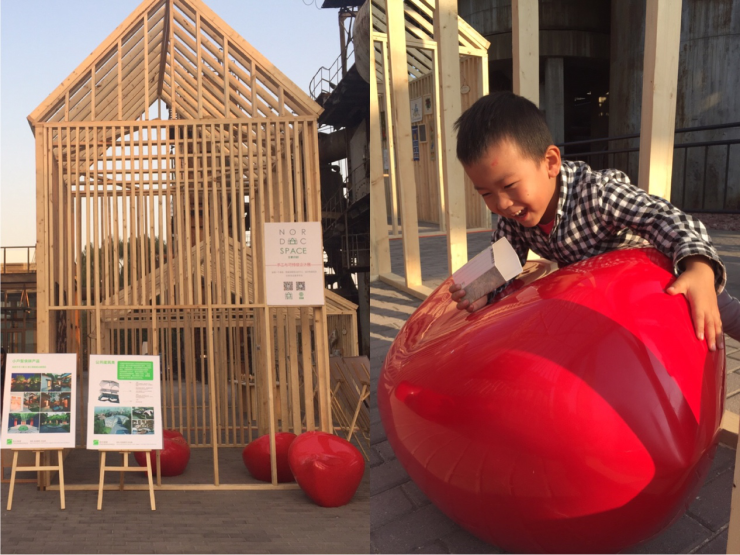（土人设计-小鸡系列作品）

关于“北欧木居”的小tips~

【北欧木居是什么】

北欧木居由北欧可持续设计协会（SSDA）策划、北京水木一方规划设计有限公司承办。由SSDA执行长、旅瑞华人、设计与品牌战略专家Soifa He策展，瑞典著名产品设计和室内建筑师Jon Eliason及其团队主导设计。

“北欧木居(Nordic Space)”是一个发现、传播和展售北欧手工、设计和建筑的线上线下北欧生活美学平台。北欧森林覆盖率70%，北欧人生活方方面面与木相关。“北欧木居（Nordic Space）——手工与可持续设计展”以木为主要元素，结合建筑与家居，传统与现代，试图还原“林子里的北欧人”的真实生活。`声明：本文由入驻焦点开放平台的作者撰写，除焦点官方账号外，观点仅代表作者本人，不代表焦点立场错误信息举报电话： 400-099-0099，邮箱：jubao@vip.sohu.com，或点此进行意见反馈，或点此进行举报投诉。`A B C D E F G H J K L M N P Q R S T W X Y Z
A - B - C - D - E
• A
• 鞍山
• 安庆
• 安阳
• 安顺
• 安康
• 澳门
• B
• 北京
• 保定
• 包头
• 巴彦淖尔
• 本溪
• 蚌埠
• 亳州
• 滨州
• 北海
• 百色
• 巴中
• 毕节
• 保山
• 宝鸡
• 白银
• 巴州
• C
• 承德
• 沧州
• 长治
• 赤峰
• 朝阳
• 长春
• 常州
• 滁州
• 池州
• 长沙
• 常德
• 郴州
• 潮州
• 崇左
• 重庆
• 成都
• 楚雄
• 昌都
• 慈溪
• 常熟
• D
• 大同
• 大连
• 丹东
• 大庆
• 东营
• 德州
• 东莞
• 德阳
• 达州
• 大理
• 德宏
• 定西
• 儋州
• 东平
• E
• 鄂尔多斯
• 鄂州
• 恩施
F - G - H - I - J
• F
• 抚顺
• 阜新
• 阜阳
• 福州
• 抚州
• 佛山
• 防城港
• G
• 赣州
• 广州
• 桂林
• 贵港
• 广元
• 广安
• 贵阳
• 固原
• H
• 邯郸
• 衡水
• 呼和浩特
• 呼伦贝尔
• 葫芦岛
• 哈尔滨
• 黑河
• 淮安
• 杭州
• 湖州
• 合肥
• 淮南
• 淮北
• 黄山
• 菏泽
• 鹤壁
• 黄石
• 黄冈
• 衡阳
• 怀化
• 惠州
• 河源
• 贺州
• 河池
• 海口
• 红河
• 汉中
• 海东
• 怀来
• I
• J
• 晋中
• 锦州
• 吉林
• 鸡西
• 佳木斯
• 嘉兴
• 金华
• 景德镇
• 九江
• 吉安
• 济南
• 济宁
• 焦作
• 荆门
• 荆州
• 江门
• 揭阳
• 金昌
• 酒泉
• 嘉峪关
K - L - M - N - P
• K
• 开封
• 昆明
• 昆山
• L
• 廊坊
• 临汾
• 辽阳
• 连云港
• 丽水
• 六安
• 龙岩
• 莱芜
• 临沂
• 聊城
• 洛阳
• 漯河
• 娄底
• 柳州
• 来宾
• 泸州
• 乐山
• 六盘水
• 丽江
• 临沧
• 拉萨
• 林芝
• 兰州
• 陇南
• M
• 牡丹江
• 马鞍山
• 茂名
• 梅州
• 绵阳
• 眉山
• N
• 南京
• 南通
• 宁波
• 南平
• 宁德
• 南昌
• 南阳
• 南宁
• 内江
• 南充
• P
• 盘锦
• 莆田
• 平顶山
• 濮阳
• 攀枝花
• 普洱
• 平凉
Q - R - S - T - W
• Q
• 秦皇岛
• 齐齐哈尔
• 衢州
• 泉州
• 青岛
• 清远
• 钦州
• 黔南
• 曲靖
• 庆阳
• R
• 日照
• 日喀则
• S
• 石家庄
• 沈阳
• 双鸭山
• 绥化
• 上海
• 苏州
• 宿迁
• 绍兴
• 宿州
• 三明
• 上饶
• 三门峡
• 商丘
• 十堰
• 随州
• 邵阳
• 韶关
• 深圳
• 汕头
• 汕尾
• 三亚
• 三沙
• 遂宁
• 山南
• 商洛
• 石嘴山
• T
• 天津
• 唐山
• 太原
• 通辽
• 铁岭
• 泰州
• 台州
• 铜陵
• 泰安
• 铜仁
• 铜川
• 天水
• 天门
• W
• 乌海
• 乌兰察布
• 无锡
• 温州
• 芜湖
• 潍坊
• 威海
• 武汉
• 梧州
• 渭南
• 武威
• 吴忠
• 乌鲁木齐
X - Y - Z
• X
• 邢台
• 徐州
• 宣城
• 厦门
• 新乡
• 许昌
• 信阳
• 襄阳
• 孝感
• 咸宁
• 湘潭
• 湘西
• 西双版纳
• 西安
• 咸阳
• 西宁
• 仙桃
• 西昌
• Y
• 运城
• 营口
• 盐城
• 扬州
• 鹰潭
• 宜春
• 烟台
• 宜昌
• 岳阳
• 益阳
• 永州
• 阳江
• 云浮
• 玉林
• 宜宾
• 雅安
• 玉溪
• 延安
• 榆林
• 银川
• Z
• 张家口
• 镇江
• 舟山
• 漳州
• 淄博
• 枣庄
• 郑州
• 周口
• 驻马店
• 株洲
• 张家界
• 珠海
• 湛江
• 肇庆
• 中山
• 自贡
• 资阳
• 遵义
• 昭通
• 张掖
• 中卫

1室1厅1厨1卫1阳台

1
2
3
4
5

0
1
2

1

1

0
1
2
3报名成功，资料已提交审核A B C D E F G H J K L M N P Q R S T W X Y Z
A - B - C - D - E
• A
• 鞍山
• 安庆
• 安阳
• 安顺
• 安康
• 澳门
• B
• 北京
• 保定
• 包头
• 巴彦淖尔
• 本溪
• 蚌埠
• 亳州
• 滨州
• 北海
• 百色
• 巴中
• 毕节
• 保山
• 宝鸡
• 白银
• 巴州
• C
• 承德
• 沧州
• 长治
• 赤峰
• 朝阳
• 长春
• 常州
• 滁州
• 池州
• 长沙
• 常德
• 郴州
• 潮州
• 崇左
• 重庆
• 成都
• 楚雄
• 昌都
• 慈溪
• 常熟
• D
• 大同
• 大连
• 丹东
• 大庆
• 东营
• 德州
• 东莞
• 德阳
• 达州
• 大理
• 德宏
• 定西
• 儋州
• 东平
• E
• 鄂尔多斯
• 鄂州
• 恩施
F - G - H - I - J
• F
• 抚顺
• 阜新
• 阜阳
• 福州
• 抚州
• 佛山
• 防城港
• G
• 赣州
• 广州
• 桂林
• 贵港
• 广元
• 广安
• 贵阳
• 固原
• H
• 邯郸
• 衡水
• 呼和浩特
• 呼伦贝尔
• 葫芦岛
• 哈尔滨
• 黑河
• 淮安
• 杭州
• 湖州
• 合肥
• 淮南
• 淮北
• 黄山
• 菏泽
• 鹤壁
• 黄石
• 黄冈
• 衡阳
• 怀化
• 惠州
• 河源
• 贺州
• 河池
• 海口
• 红河
• 汉中
• 海东
• 怀来
• I
• J
• 晋中
• 锦州
• 吉林
• 鸡西
• 佳木斯
• 嘉兴
• 金华
• 景德镇
• 九江
• 吉安
• 济南
• 济宁
• 焦作
• 荆门
• 荆州
• 江门
• 揭阳
• 金昌
• 酒泉
• 嘉峪关
K - L - M - N - P
• K
• 开封
• 昆明
• 昆山
• L
• 廊坊
• 临汾
• 辽阳
• 连云港
• 丽水
• 六安
• 龙岩
• 莱芜
• 临沂
• 聊城
• 洛阳
• 漯河
• 娄底
• 柳州
• 来宾
• 泸州
• 乐山
• 六盘水
• 丽江
• 临沧
• 拉萨
• 林芝
• 兰州
• 陇南
• M
• 牡丹江
• 马鞍山
• 茂名
• 梅州
• 绵阳
• 眉山
• N
• 南京
• 南通
• 宁波
• 南平
• 宁德
• 南昌
• 南阳
• 南宁
• 内江
• 南充
• P
• 盘锦
• 莆田
• 平顶山
• 濮阳
• 攀枝花
• 普洱
• 平凉
Q - R - S - T - W
• Q
• 秦皇岛
• 齐齐哈尔
• 衢州
• 泉州
• 青岛
• 清远
• 钦州
• 黔南
• 曲靖
• 庆阳
• R
• 日照
• 日喀则
• S
• 石家庄
• 沈阳
• 双鸭山
• 绥化
• 上海
• 苏州
• 宿迁
• 绍兴
• 宿州
• 三明
• 上饶
• 三门峡
• 商丘
• 十堰
• 随州
• 邵阳
• 韶关
• 深圳
• 汕头
• 汕尾
• 三亚
• 三沙
• 遂宁
• 山南
• 商洛
• 石嘴山
• T
• 天津
• 唐山
• 太原
• 通辽
• 铁岭
• 泰州
• 台州
• 铜陵
• 泰安
• 铜仁
• 铜川
• 天水
• 天门
• W
• 乌海
• 乌兰察布
• 无锡
• 温州
• 芜湖
• 潍坊
• 威海
• 武汉
• 梧州
• 渭南
• 武威
• 吴忠
• 乌鲁木齐
X - Y - Z
• X
• 邢台
• 徐州
• 宣城
• 厦门
• 新乡
• 许昌
• 信阳
• 襄阳
• 孝感
• 咸宁
• 湘潭
• 湘西
• 西双版纳
• 西安
• 咸阳
• 西宁
• 仙桃
• 西昌
• Y
• 运城
• 营口
• 盐城
• 扬州
• 鹰潭
• 宜春
• 烟台
• 宜昌
• 岳阳
• 益阳
• 永州
• 阳江
• 云浮
• 玉林
• 宜宾
• 雅安
• 玉溪
• 延安
• 榆林
• 银川
• Z
• 张家口
• 镇江
• 舟山
• 漳州
• 淄博
• 枣庄
• 郑州
• 周口
• 驻马店
• 株洲
• 张家界
• 珠海
• 湛江
• 肇庆
• 中山
• 自贡
• 资阳
• 遵义
• 昭通
• 张掖
• 中卫• 手机• 分享
• 设计
免费设计
• 计算器
装修计算器
• 入驻
合作入驻
• 联系
联系我们
• 置顶
返回顶部